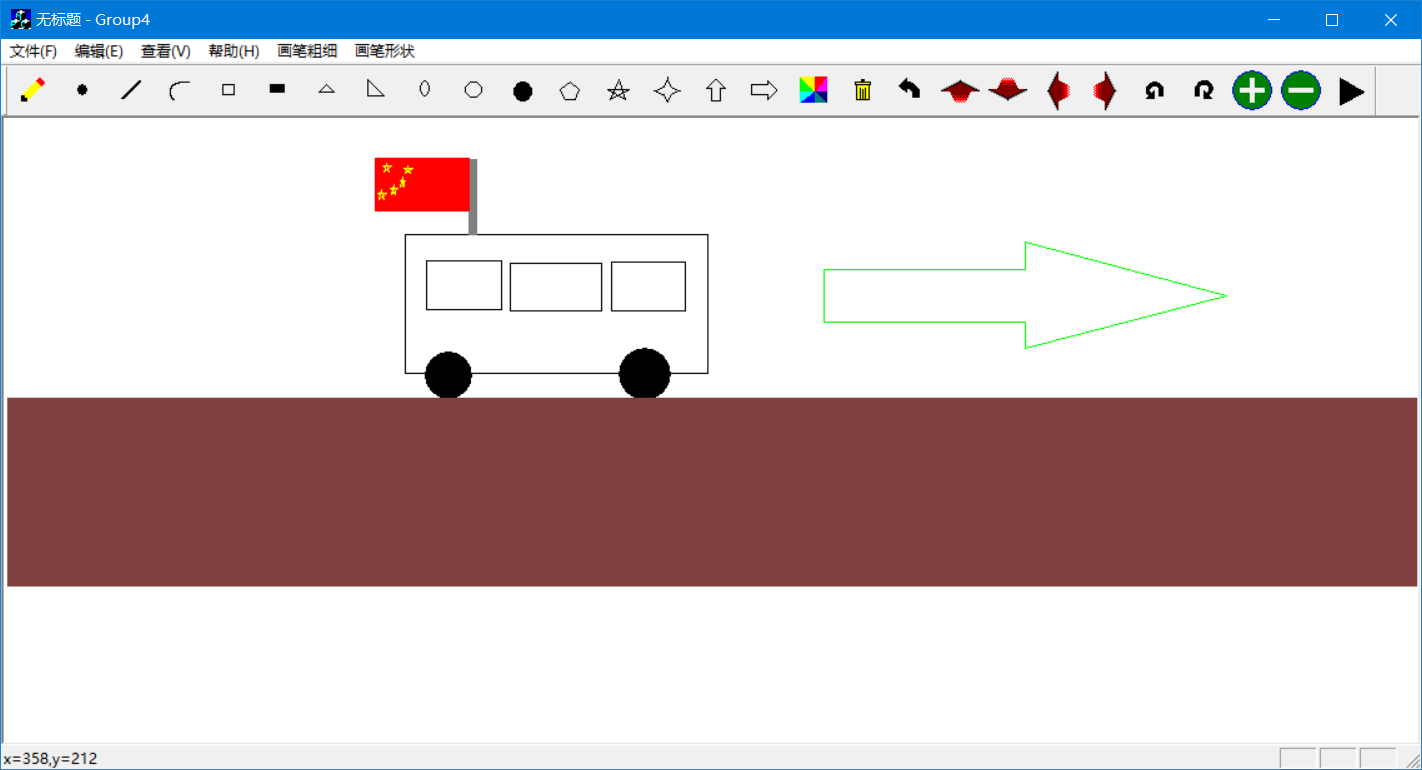# 基于MFC和二维变换的画图软件

## 设 计

“基于二维复合变换的动画制作软件”的设计中包括以下几个部分：(1) 程序结构设计，(2)鼠标消息映射，(3) 图形绘制实现，(4) 图形变换,（5）动画扩展实现,（6）信息保存,(7)程序交互设计。

### 1 程序总体结构

#### 1.1 总体结构设计

##### 1.1.2 变换设计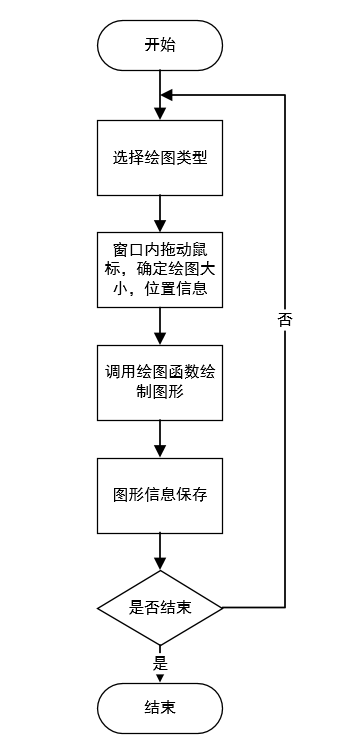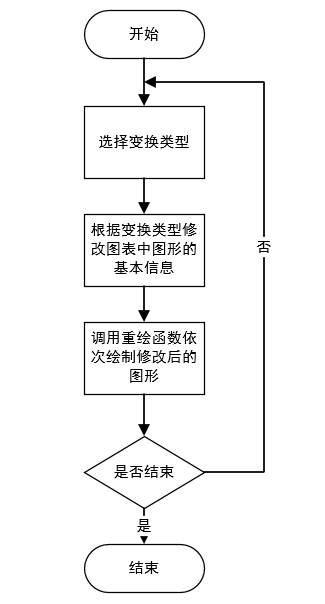### 2 程序实现

#### 2.2 图形绘制实现

##### 2.2.3 等腰和直角三角形

P1 (pStart.x+pEnd.x)/2,pStart.y);
P2 (pStart.x,pEnd.y);
P3 (pEnd.x,pEnd.y);

P1 (pStart.x,pStart.y);
P2 (pEnd.x,pEnd.y);
P3 (pStart.x,pEnd.y);

##### 2.2.7 左箭头

P1 (pStart.x, pStart.y-dy/2);
P2 (pStart.x+dx/2),pStart.y);
P3 (pStart.x+dx/2), pStart.y-dy/4);
P4 (pEnd.x, pStart.y-dy/4);
P5 (pEnd.x, pStart.y-3*dy/4);
P6 (pStart.x+dx/2), pStart.y-3*dy/4);
P7 (pStart.x+dx/2),pEnd.y);

##### 2.2.8 上箭头

P1 (pStart.x, pStart.y-dy0/2);
P2 ( (pStart.x+dx0/2,pStart.y);
P3 (pEnd.x, pStart.y-dy0/2);
P4 (pStart.x+3*dx0/4, pStart.y-dy0/2);
P5 (pStart.x+3*dx0/4,pEnd.y);
P6 (pStart.x+dx0/4,pEnd.y);
P7 (pStart.x+dx0/4, pStart.y-dy0/2);

##### 2.2.9 五角星

P1(pStart.x+pEnd.x)/2),pStart.y);
P2(pStart.x+RX*(sin(72*pi/180)cos(54*pi/180))/2/sin(72*pi/180)),pEnd.y);
P3(pEnd.x, pStart.y+RY*(1-cos(72*pi/180))/(1+sin(54*pi/180)));
P4(pStart.x, pStart.y+RY*(1-cos(72*pi/180))/(1+sin(54*pi/180)));
P5(pStart.x+RX*(sin(72*pi/180)+cos(54*pi/180))/2/sin(72*pi/180),pEnd.y);

##### 2.2.10 五边形

P1 (pStart.x+pEnd.x)/2,pStart.y);
P2 (pStart.x,(pEnd.y-pStart.y)*0.41+pStart.y);
P3 (pStart.x+(pEnd.x - pStart.x)*0.19,pEnd.y);
P4 (pStart.x+(pEnd.x - pStart.x)*0.81,pEnd.y);
P5 (pEnd.x, (pEnd.y-pStart.y)*0.41+pStart.y);

##### 2.2.11 四角星

P1 ((pStart.x+pEnd.x)/2,pStart.y)
P2 (pStart.x+(pEnd.x-pStart.x)*3/8,(pEnd.y-pStart.y)* 3/8+pStart.y);
P3 (pStart.x,(pStart.y+pEnd.y)/2);
P4 (pStart.x+(pEnd.x-pStart.x)* 3/8,(pEnd.y-pStart.y)*6/8+pStart.y);
P5 ((pStart.x + pEnd.x)/2),pEnd.y);
P6 (pStart.x+(pEnd.x-pStart.x)* 6/8,(pEnd.y-pStart.y)*6/8+pStart.y);
P7 (pEnd.x,(pStart.y+pEnd.y)/2);
P8 (pStart.x+(pEnd.x-pStart.x)*6/8,(pEnd.y-pStart.y)*3/8+pStart.y);

#### 2.3 图形变换实现

##### 2.4.2 自定义点表结构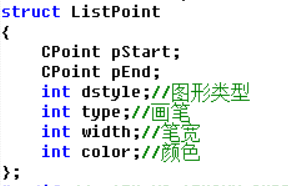##### 2.4.3 运动时间设置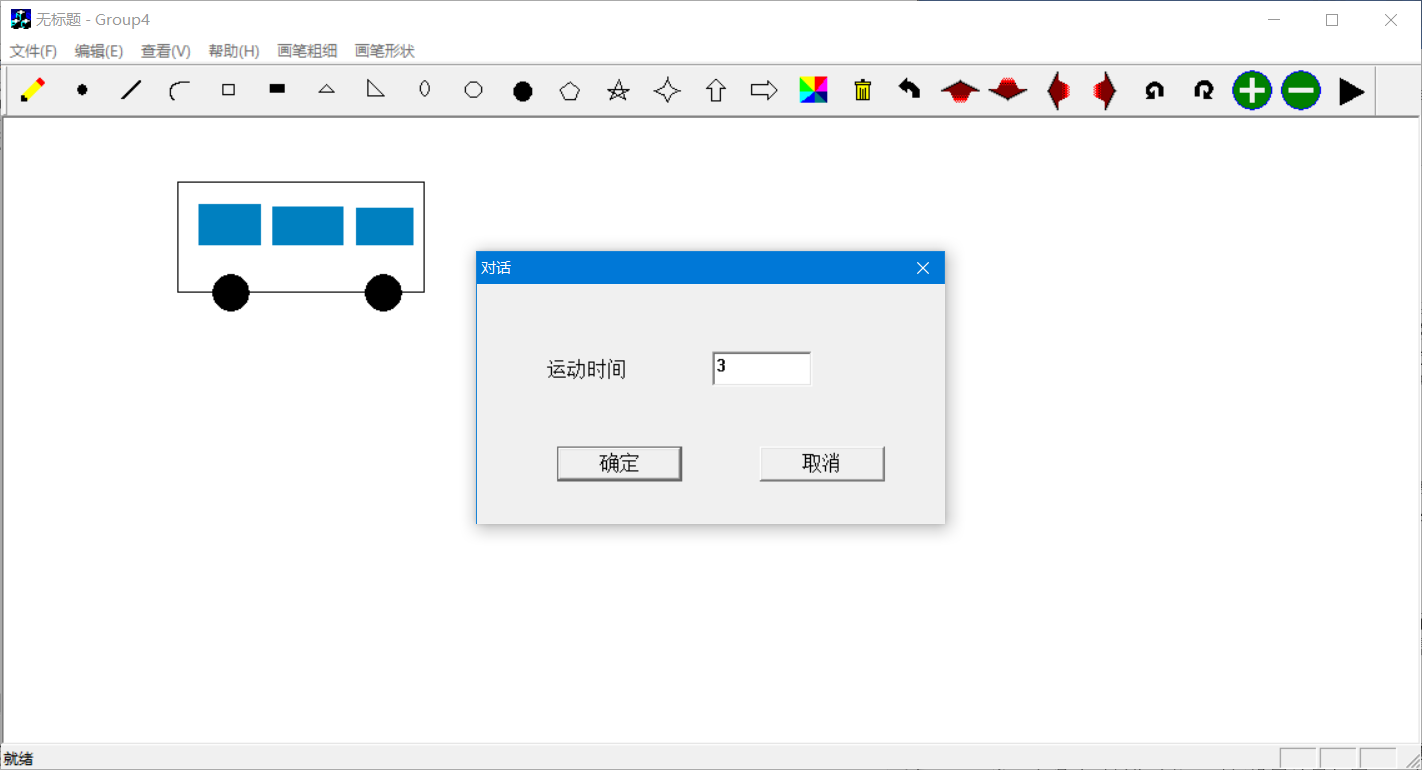##### 2.5.1 绘图类型选择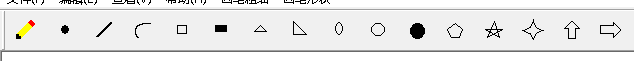##### 2.5.2 画笔颜色选择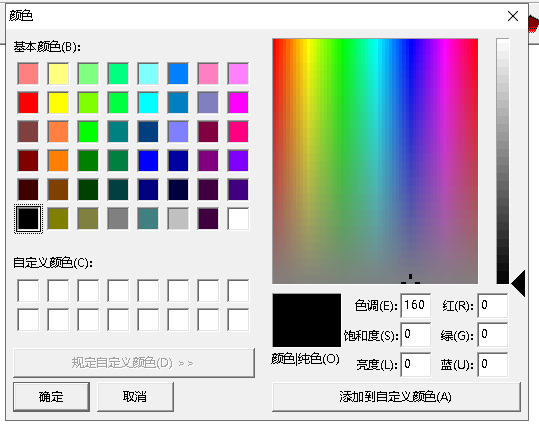### 3 程序运行效果

#### 3.1 基本图形实现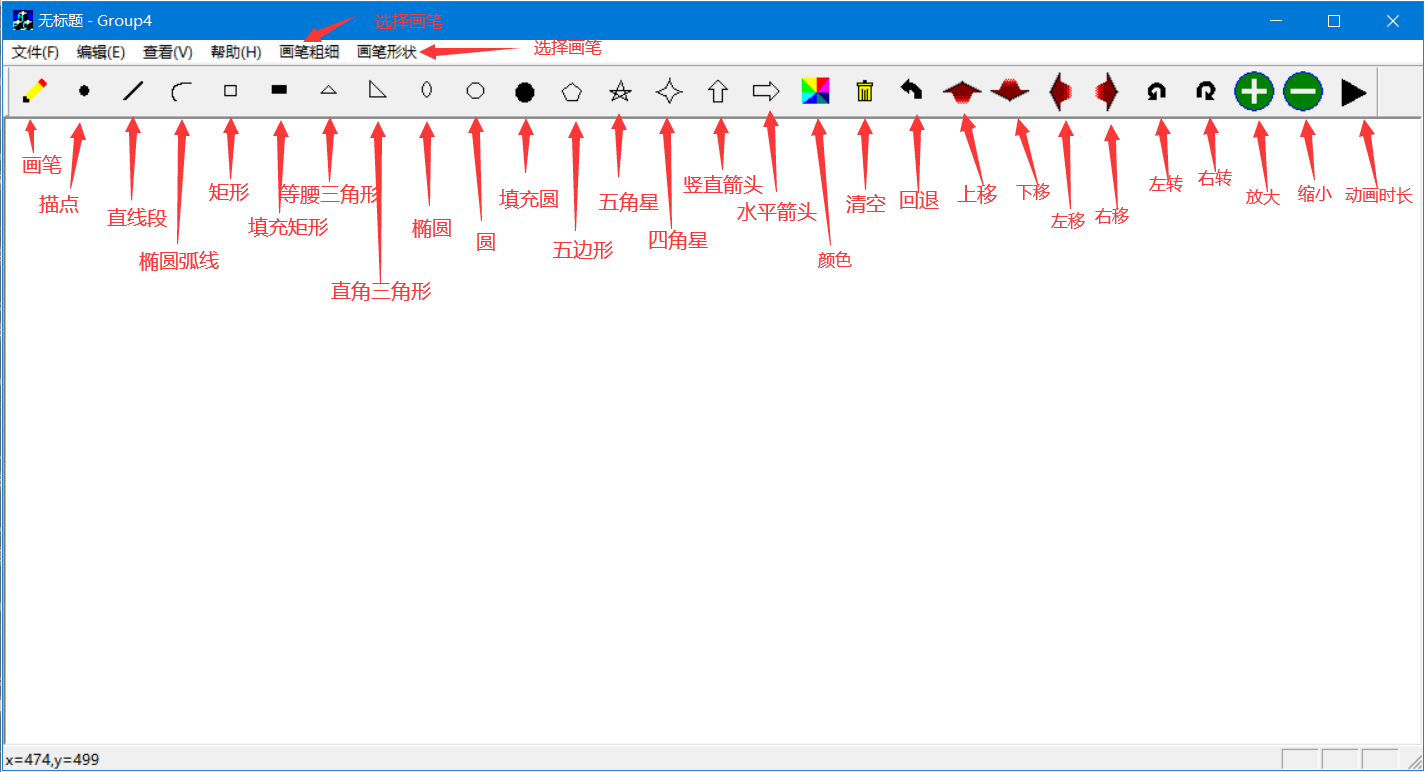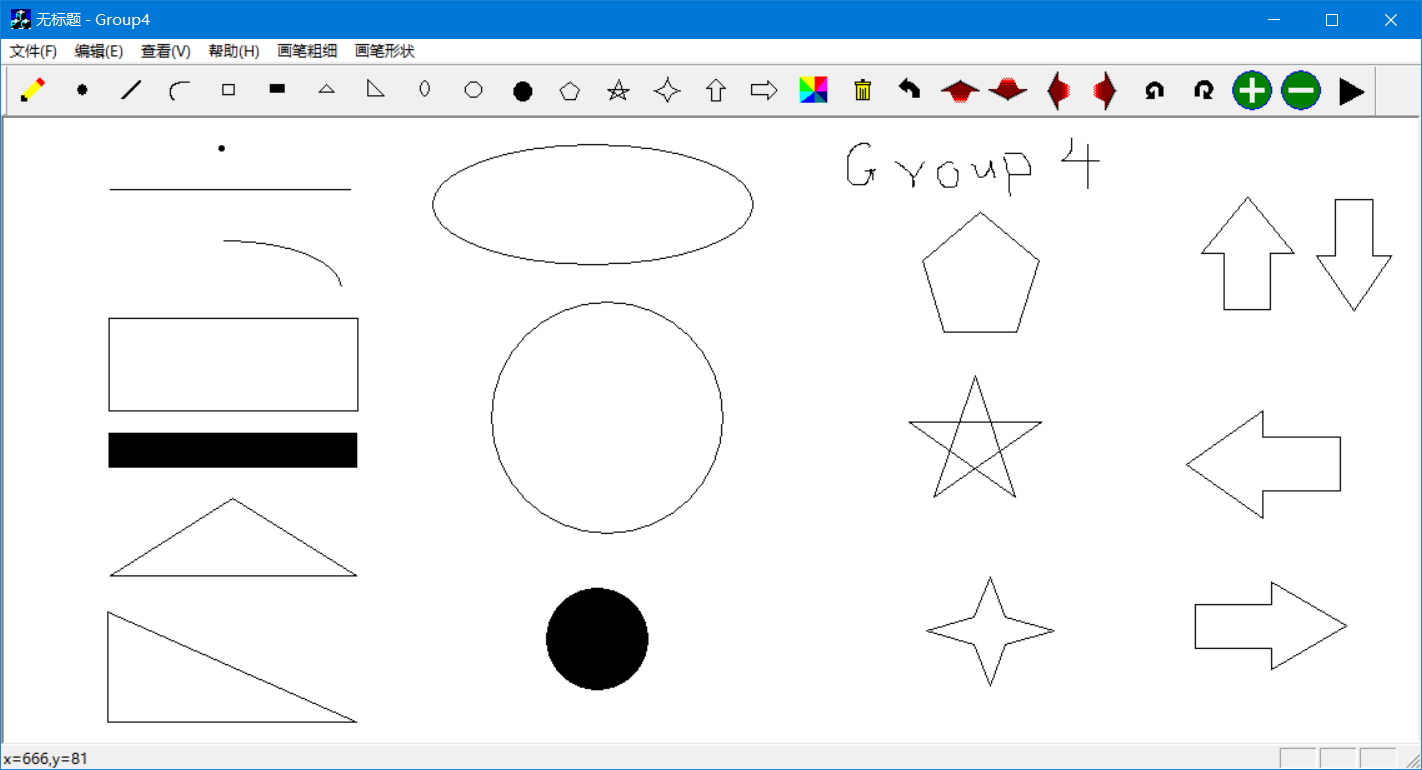#### 3.2 组合复杂图形以及整体变换